# Scripting Physical Environmentsbacklinks... CindyLab Table of Contents English 日本語

## Scripting Physical Environments

There are many ways in which CindyScript can be used to enhance the possibilities of CindyLab. We will present a few of them here. On the one hand, CindyScript provides several special operators that have been implemented for use with CindyScript (for instance, an oscilloscope for viewing physical magnitudes and a statement for plotting force fields). On the other hand, CindyScript has direct access to most of the parameters that are relevant for physics simulations. Moreover, CindyScript can enable or disable physical functionalities of CindyLab objects. In this way one can implement machine-like behavior in CindyLab scenarios. We will give several examples here that use CindyScript together with CindyLab.

### The Oscilloscope

The statement drawcurves(position,list_of_values) generates a region in the geometric view in which the development of magnitudes can be shown. Here position is the position at which the oscilloscope is drawn and list_of_values is a CindyScript list that contains several magnitudes to be represented graphically. The simplest use of this operator is presented by the following program:

drawcurves((0,0),[B.x,B.y])


Here the x and y coordinates of point B are shown. If B is a point moving around a sun, the picture might look like the one below.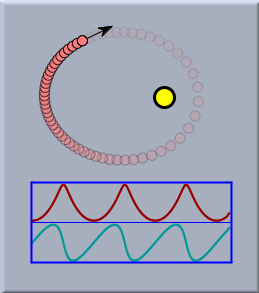Curved plots of planetary motion

By default, the display range of the curve is automatically adapted to the range of values. The drawcurves(...) operator supports many different modifiers that can be usedto set background colors, curve colors, display texts, as well as many additional parameters for drawings. You will find a detailed description in the CindyScript reference manual. The picture below shows the use of the drawcurves operator applied to a coupled harmonic oscillator.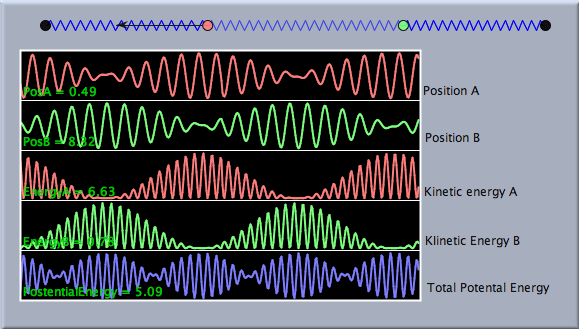The movement of a coupled oscillator

Here many modifiers were used in order to get the desired color behavior and the text display. The example was constructed using the following CindyScript program:

linecolor((1,1,1));
drawcurves((-7,-3),
textcolor((1,1,1));  (A.x,B.x,A.ke,B.ke,a.pe+b.pe+c.pe),
height->50,
color->(1,1,1),
back->(0,0,0),
backalpha->1,showranges->true,
range->"peek",width->400,
colors->[
[1,0.5,0.5],
[0.5,1,0.5],
[1,0.5,0.5],
[0.5,1,0.5],
[0.5,0.5,1]],
texts->[
"PosA = "+ A.x,
"PosB = "+B.x,
"EnergyA = "+A.ke,
"EnergyB = "+B.ke,
"PotentialEnergy = "+(a.pe+b.pe+c.pe)
]);


### Force Fields

Another CindyScript operator that was designed to support CindyLab is the drawforces operator. With this operator one can visualize the direction and strength of forces all over the drawing area. To accomplish this, a virtual probe particle moves across the screen, measuring the forces at different points. If one simply writes in CindyScript the line

drawforces()


then all gravitational forces, Coulomb forces, ball interactions, etc. are measured and the flux is drawn. The probe particle is standardized to have mass = 1, charge = 1, and radius = 1. If, for instance, two charged particles are present with opposite charges, then the drawforces() operator causes the following flux picture: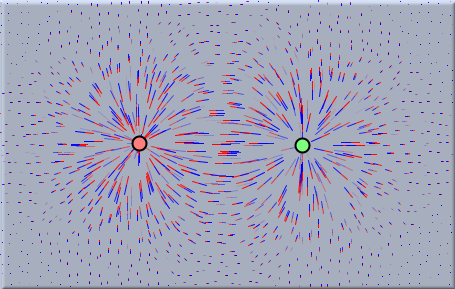A simple electrostatic field

Like the drawcurves() operator, the drawforces() operator supports many modifiers. They are explained in detail in the CindyScript reference. Here we just present a slightly more advanced example.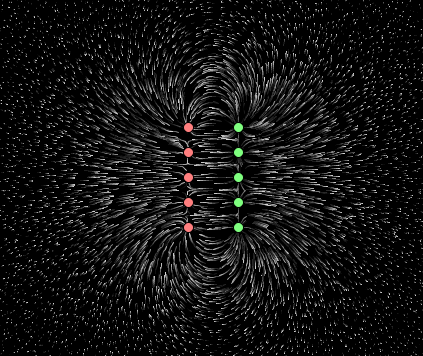Another simple electrostatic field

The code fragment that generated this flux picture uses several modifiers.

drawforces(stream->true,
color->(1,1,1),
factor->3,
resolution->5)


In particular, the stream->true statement replaces the usual display of force fields with small needles with little streamlets that give a more accurate picture of the flux.

### Programming CindyLab Scenarios

Since CindyScript can influence all the parameters of CindyLab, it is also easy to implement complicated behavior. As an example, we present here an implementation of a simple table tennis game. First, a few bouncers are used to draw the boundary of the table. Another bouncer is constructed geometrically that is bound to a point so that it can be freely moved like a table tennis paddle. Finally, a table tennis ball is added as a particle with velocity. So far, the construction makes use only of CindyLab and Cinderella. The situation is already quite functional. One can indeed play table tennis. However, if the ball leaves the screen, it will be lost forever.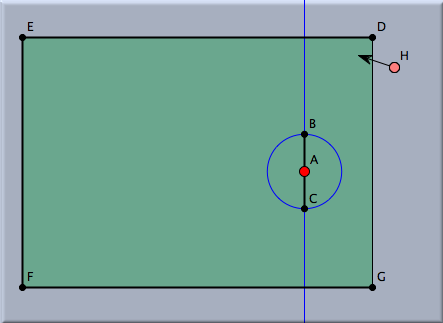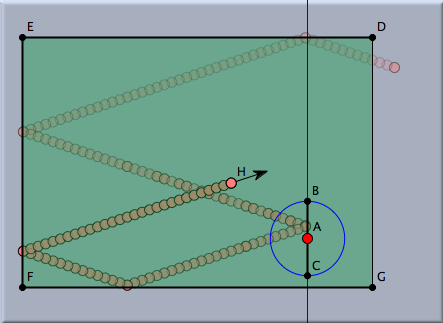Construction of a table tennis game A lost ball

This is the point at which CindyScript comes into play. With CindyScript one can detect whether the ball is leaving the screen and react to such an event. The following program detects the ball leaving the screen with the code line if(H.x>D.x+9,.... If this happens, a new point with random position, random speed, and random color is inserted at the end of the table. In addition, a counter is used to count how many balls have been lost.

if(H.x>D.x+9,
H.x=F.x;
r=random();
H.y=(r*D.y+(1-r)*G.y);
w=random()*pi/2+3*pi/4;
H.vx=-cos(w)*.4;
H.vy=sin(w)*.4;
H.color=(hue(random()));
count=count+1;
);
drawtext((-1,-3),"Missed balls: "+count,size->20);


In order to initialize the counter, there must also be one additional line of code in the simulation start tag that sets the counter to zero.

count=0;


The picture below shows a snapshot that was taken during a game. Observe how the yellow ball leaves the game and the blue ball is thrown in.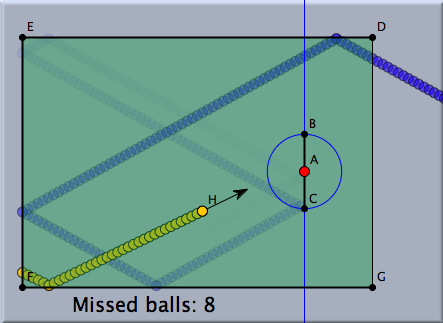Scripting the table tennis game

Contributors to this page: Richter , Kortenkamp , Kohler and Kramer .
Page last modified on Sunday 04 of September, 2011 [18:32:10 UTC] by Richter.